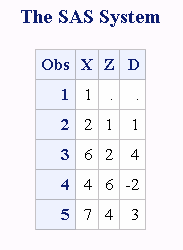# DIF Function

Returns differences between an argument and its nth lag.

 Category: Special

## Syntax

DIF<n> (argument)

### Required Argument

#### argument

specifies a numeric constant, variable, or expression.

### Optional Argument

#### n

specifies the number of lags.

## Details

The DIF functions, DIF1, DIF2, ..., DIF100, return the first differences between the argument and its nth lag. DIF1 can also be written as DIF. DIFn is defined as DIFn(x)=x-LAGn(x).
For details about storing and returning values from the LAGn queue, see the LAG function.

## Comparisons

The function DIF2(X) is not equivalent to the second difference DIF(DIF(X)).

## Example

This example demonstrates the difference between the LAG and DIF functions.
```data two;
input X @@;
Z=lag(x);
D=dif(x);
datalines;
1 2 6 4 7
;
proc print data=two;
run;```
Difference between the DIF and LAG Functions